# FrostImageFilter.cxx¶

This example illustrates the use of the otb::FrostImageFilter. This filter belongs to the family of the edge-preserving smoothing filters which are usually used for speckle reduction in radar images.

This filter uses a negative exponential convolution kernel. The output of the filter for pixel p is: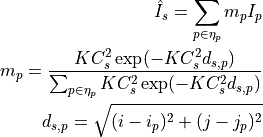where:

•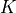: the decrease coefficient

•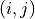: the coordinates of the pixel inside the region defined by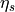•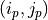: the coordinates of the pixels belonging to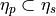•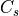: the variation coefficient computed over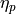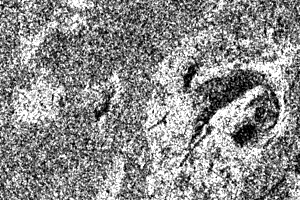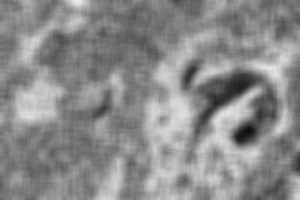Result of applying the FrostImageFilter to a SAR image.

Example usage:

./FrostImageFilter Input/GomaSmall.png Output/GomaSmallFrostFiltered.png 5 0.1


Example source code (FrostImageFilter.cxx):

#include "otbFrostImageFilter.h"

#include "otbImage.h"
#include "otbImageFileWriter.h"

int main(int argc, char* argv[])
{

if (argc != 5)
{
std::cerr << "Usage: " << argv << " inputImageFile ";
std::cerr << " outputImageFile radius deramp" << std::endl;
return EXIT_FAILURE;
}

using PixelType       = unsigned char;
using InputImageType  = otb::Image<PixelType, 2>;
using OutputImageType = otb::Image<PixelType, 2>;

// The filter can be instantiated using the image types defined previously.
using FilterType = otb::FrostImageFilter<InputImageType, OutputImageType>;
using WriterType = otb::ImageFileWriter<OutputImageType>;

FilterType::Pointer filter = FilterType::New();

WriterType::Pointer writer = WriterType::New();
writer->SetInput(filter->GetOutput());

// The image obtained with the reader is passed as input to the FrostImageFilter

// The method SetRadius() defines the size of the window to
// be used for the computation of the local statistics. The method
// SetDeramp() sets the K coefficient.